Algebra 2 4-1 Guided Practice: Quadratic Functions and Transformations
starstarstarstarstarstarstarstarstarstar
by Matthew Richardson
| 11 Questions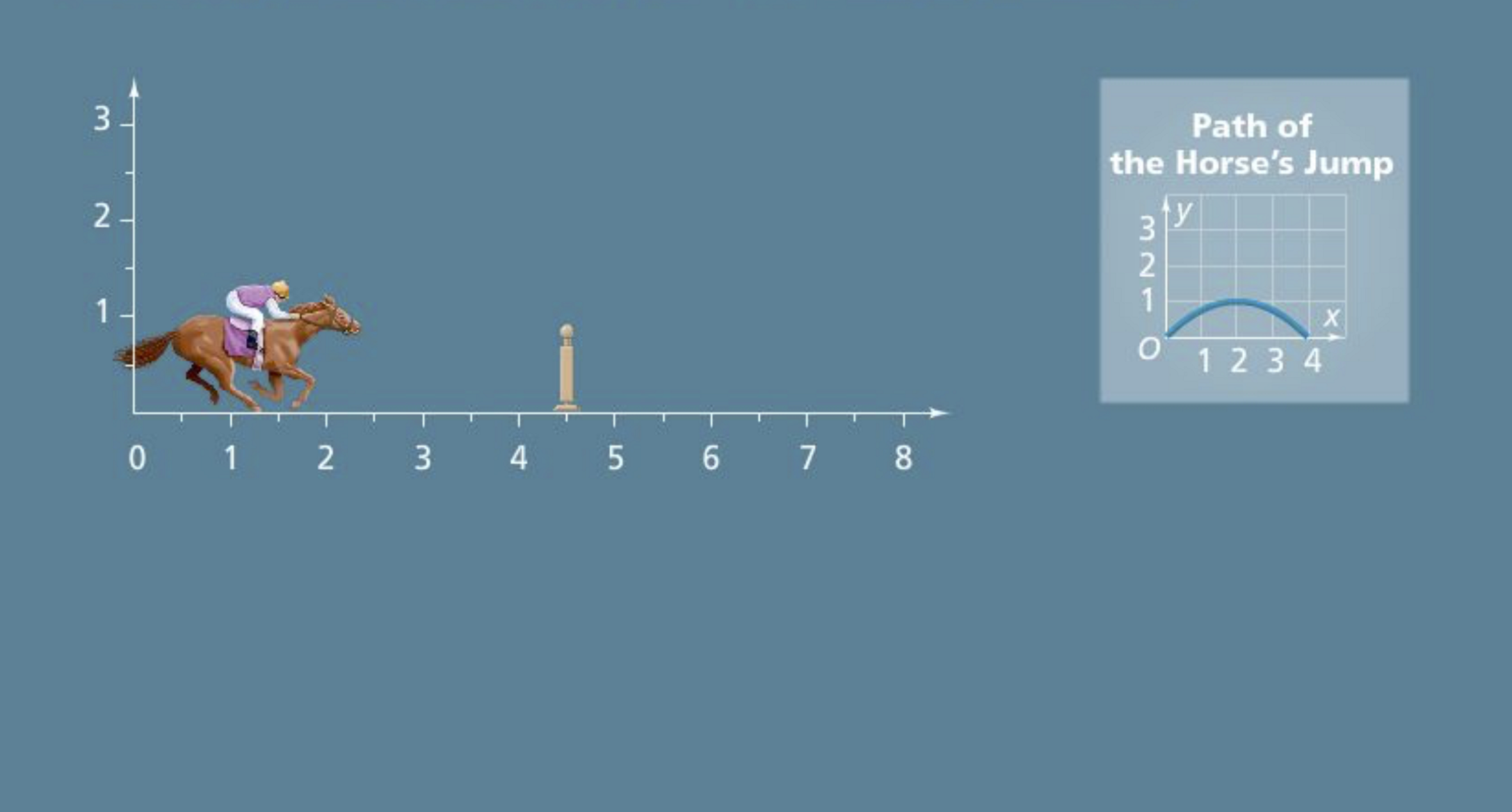a
1
Solve It! In the computer game Steeplechase, you press the "jump" button and the horse makes the jump shown. The highest part of the jump must be directly above the fence or you lose time.
1
10
Solve It! Where should this horse be when you press "jump"?
at 3.5 on the horizontal axis
at 2.5 on the horizontal axis
at 3 on the horizontal axis
at 4 on the horizontal axis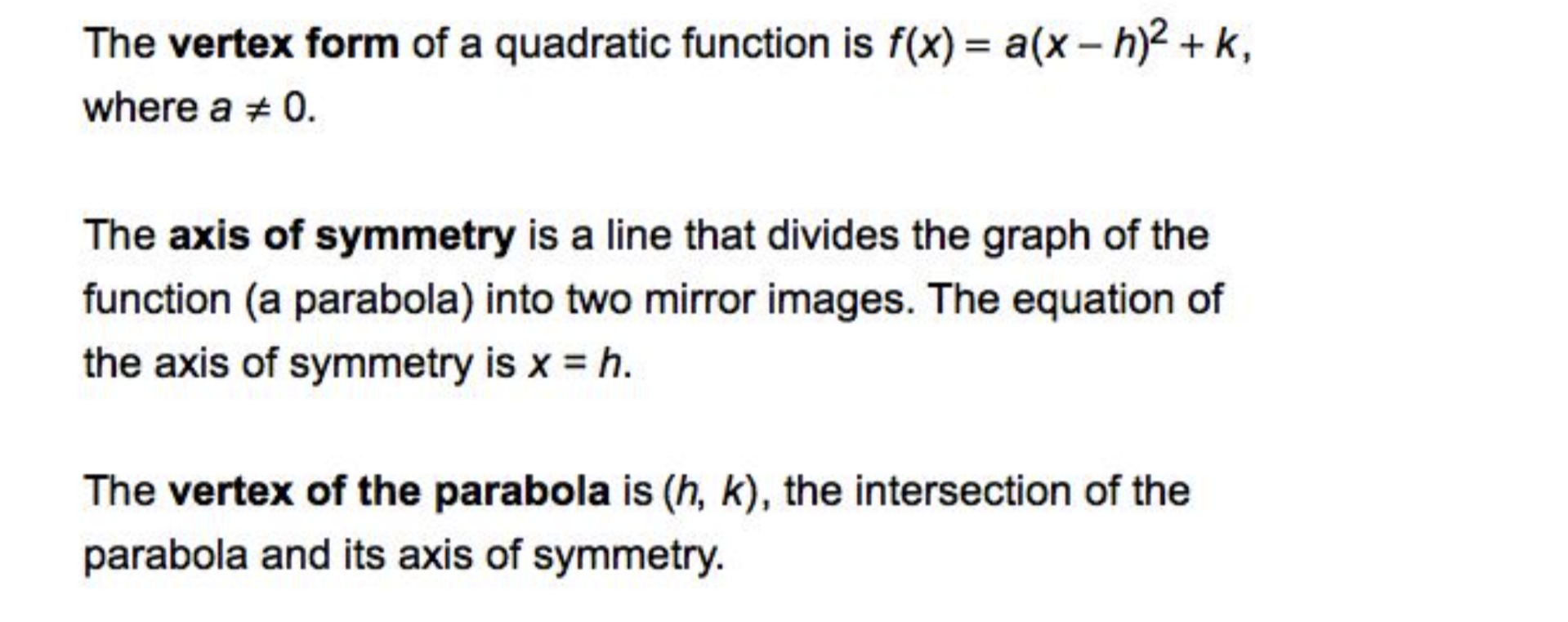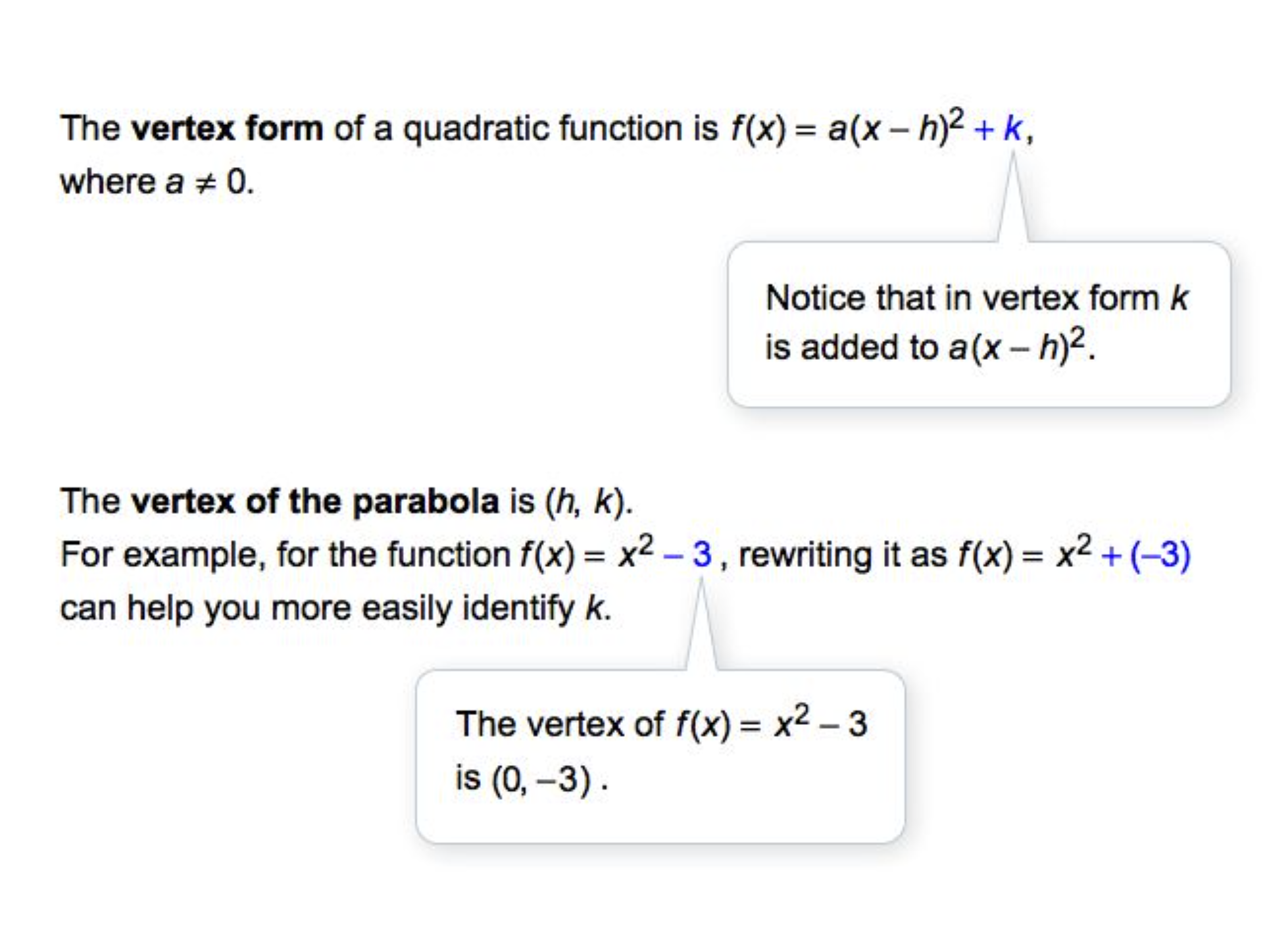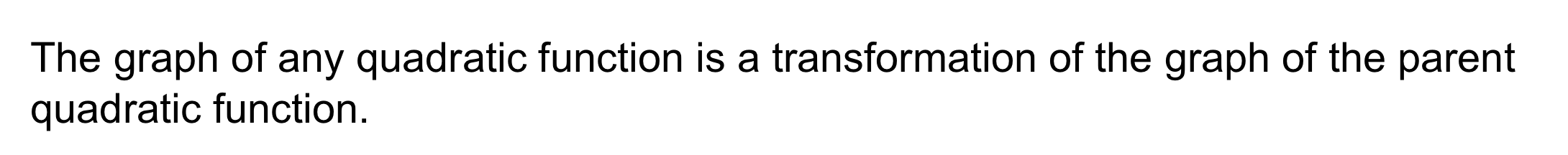2
20
Problem 1 Got It? What is the graph of the function? Complete the table of values and graph the function on the canvas. Use colors other than black.

3
10
Problem 1 Got It? Graph the parent quadratic function and its transformation on the same plane. Zoom and pan your graph to establish an appropriate viewing window. Consider the relationship between the two functions.
After graphing with the Desmos utility, you may edit your response to the previous item if needed.
4
10
Problem 1 Got It? Reasoning: What can you say about the graph of the function below if a is a negative number?

The graph is a parabola that opens downward.
The graph is a parabola that opens upward.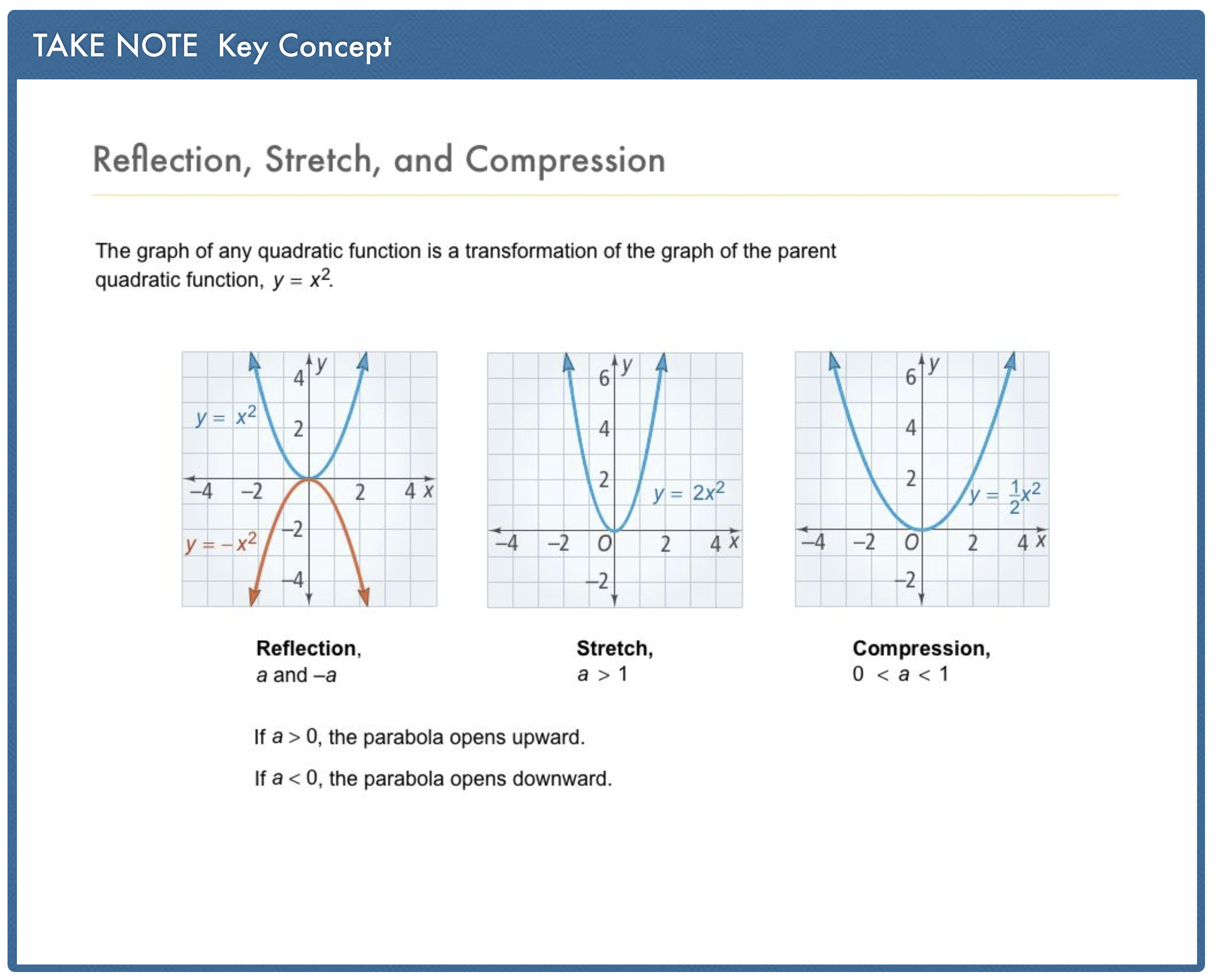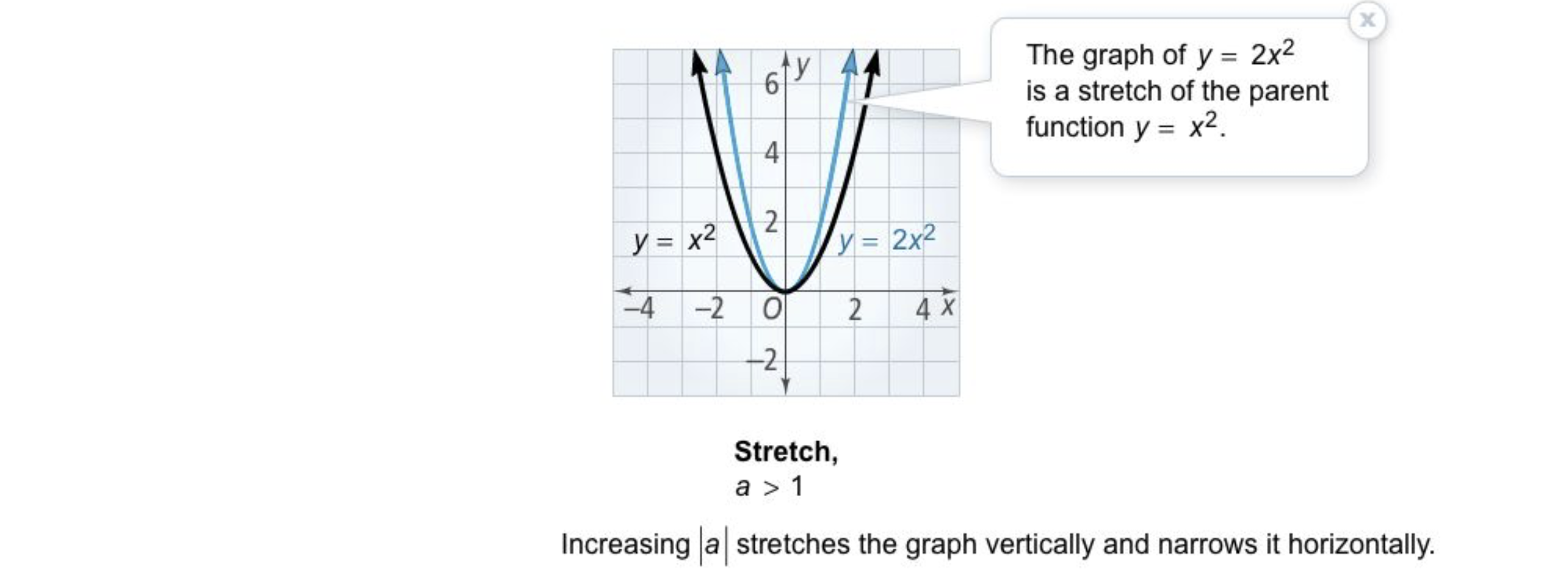5
20
Problem 2 Got It? Sketch a graph the function on the canvas. Include relevant graph detail: label axes, indicate units and scale on both axes, and use arrows to represent end behavior as appropriate.

6
10
Problem 2 Got It? How is the graph of g(x) = + 3 in the previous item a translation of the parent function f(x) = ?
g(x) is f(x) translated right 3 units
g(x) is f(x) translated down 3 units
g(x) is f(x) translated left 3 units
g(x) is f(x) translated up 3 units
7
20
Problem 2 Got It? Sketch a graph the function on the canvas. Include relevant graph detail: label axes, indicate units and scale on both axes, and use arrows to represent end behavior as appropriate.

8
10
Problem 2 Got It? How is the graph of h(x) = (x + 1)² in the previous item a translation of the parent function f(x) = ?
h(x) is f(x) translated left 1 unit
h(x) is f(x) translated down 1 unit
h(x) is f(x) translated right 1 unit
h(x) is f(x) translated up 1 unit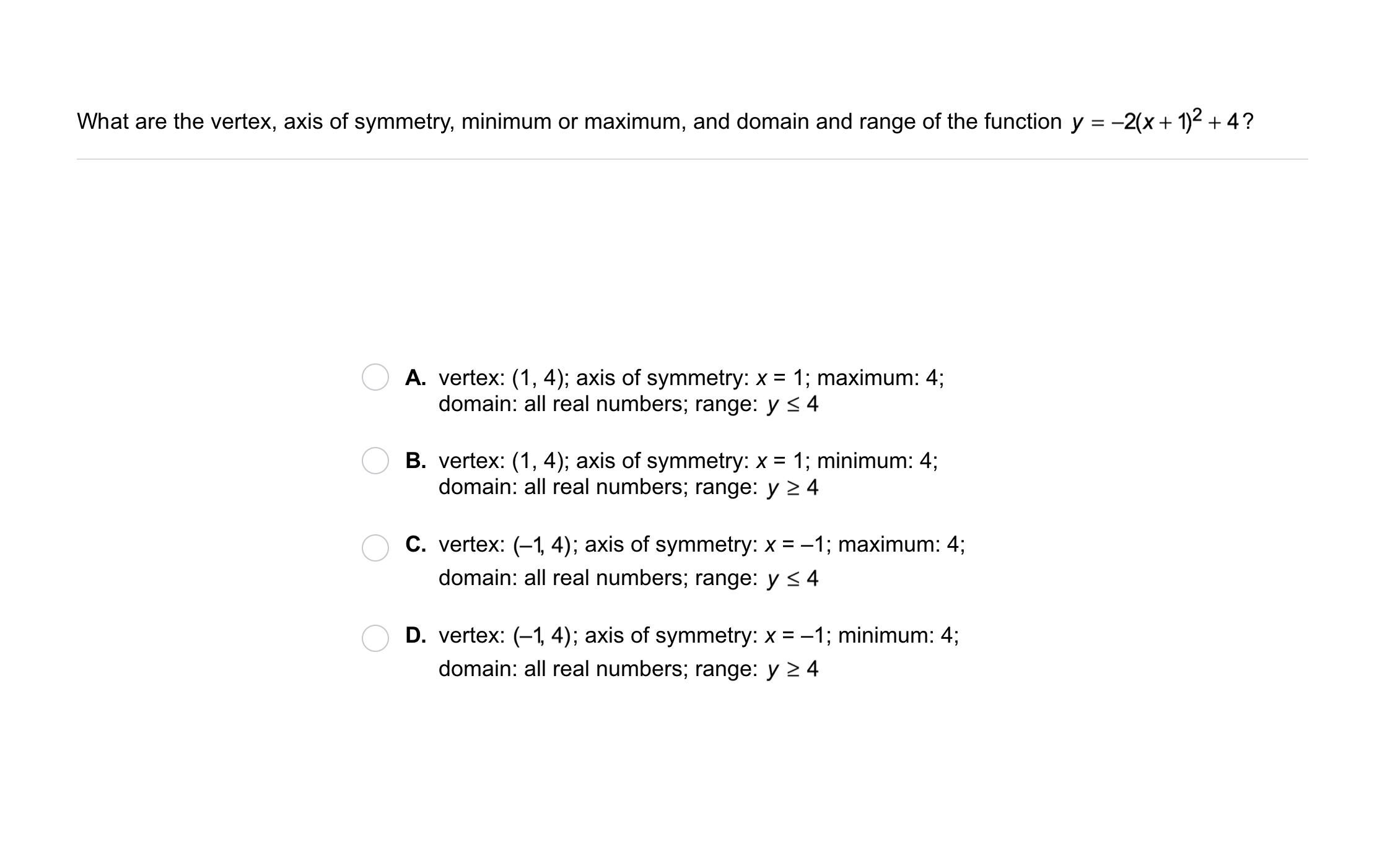9
9
10
Problem 3 Got It?
A
B
C
D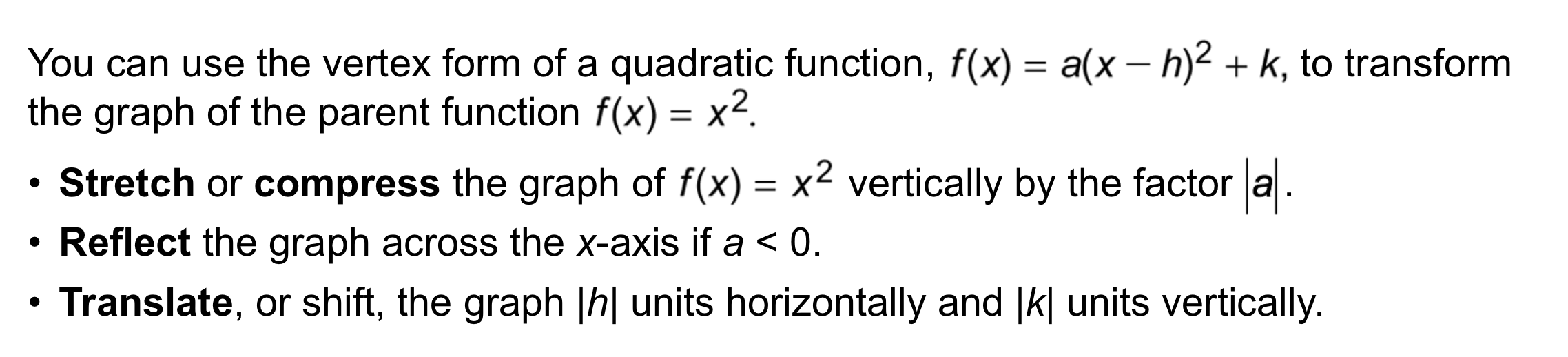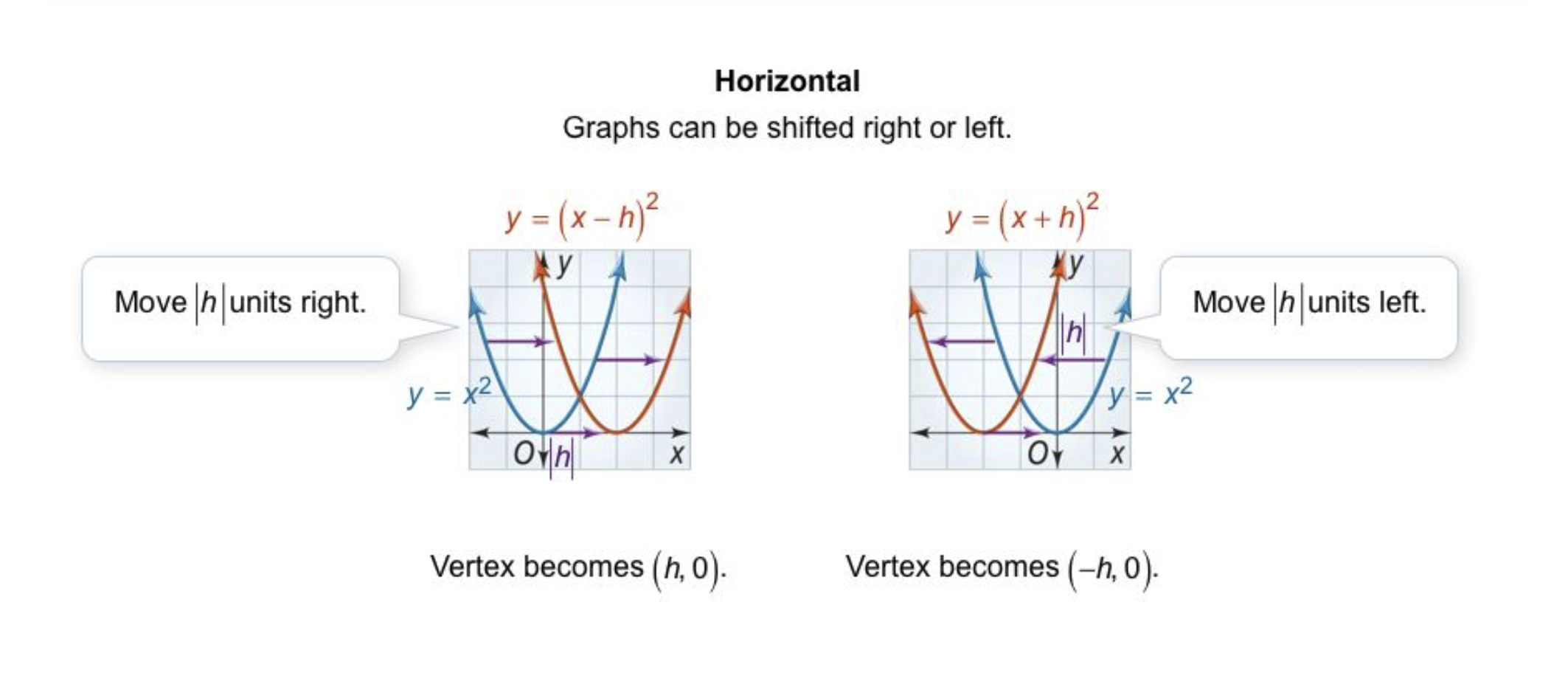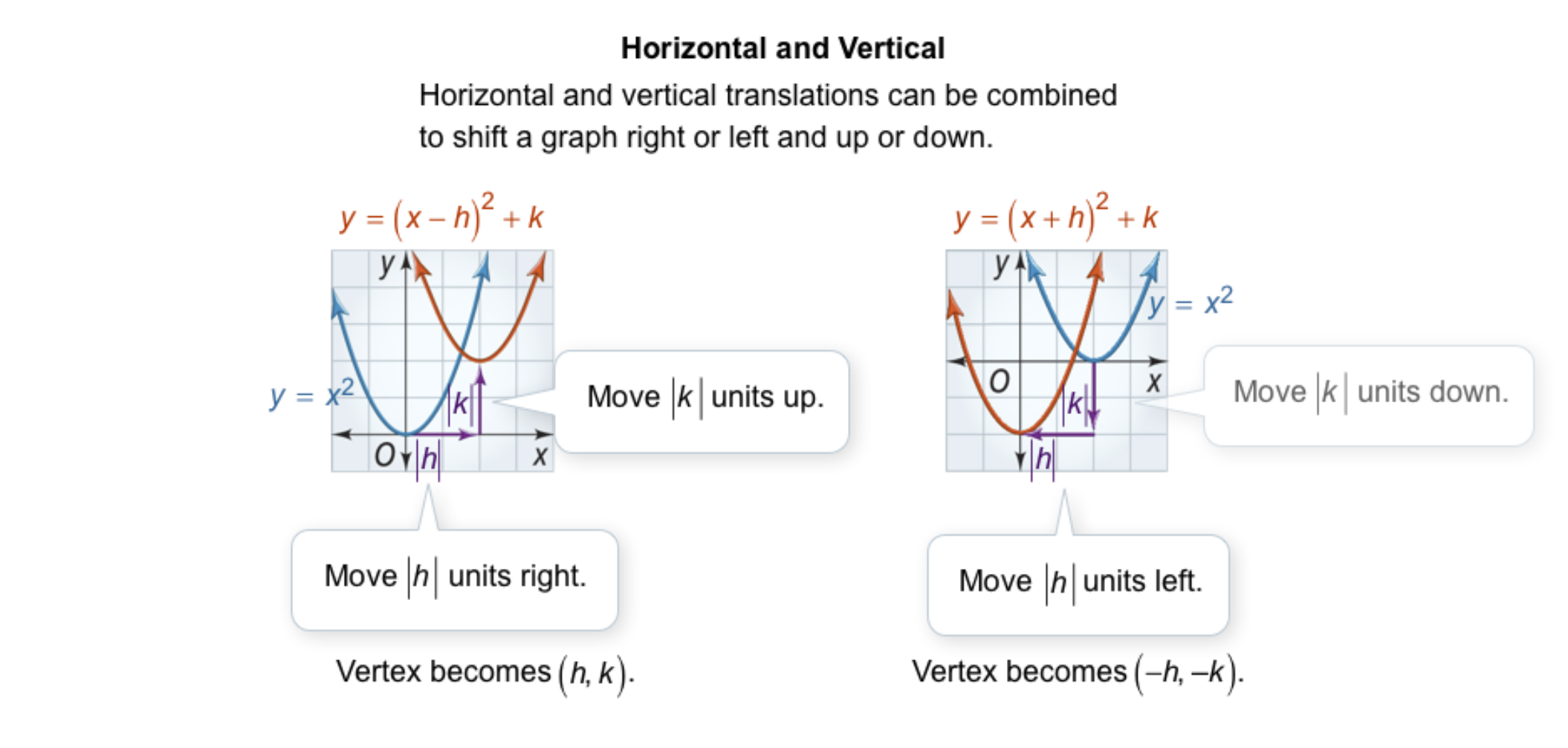10
10
10
Problem 4 Got It?
A
B
C
D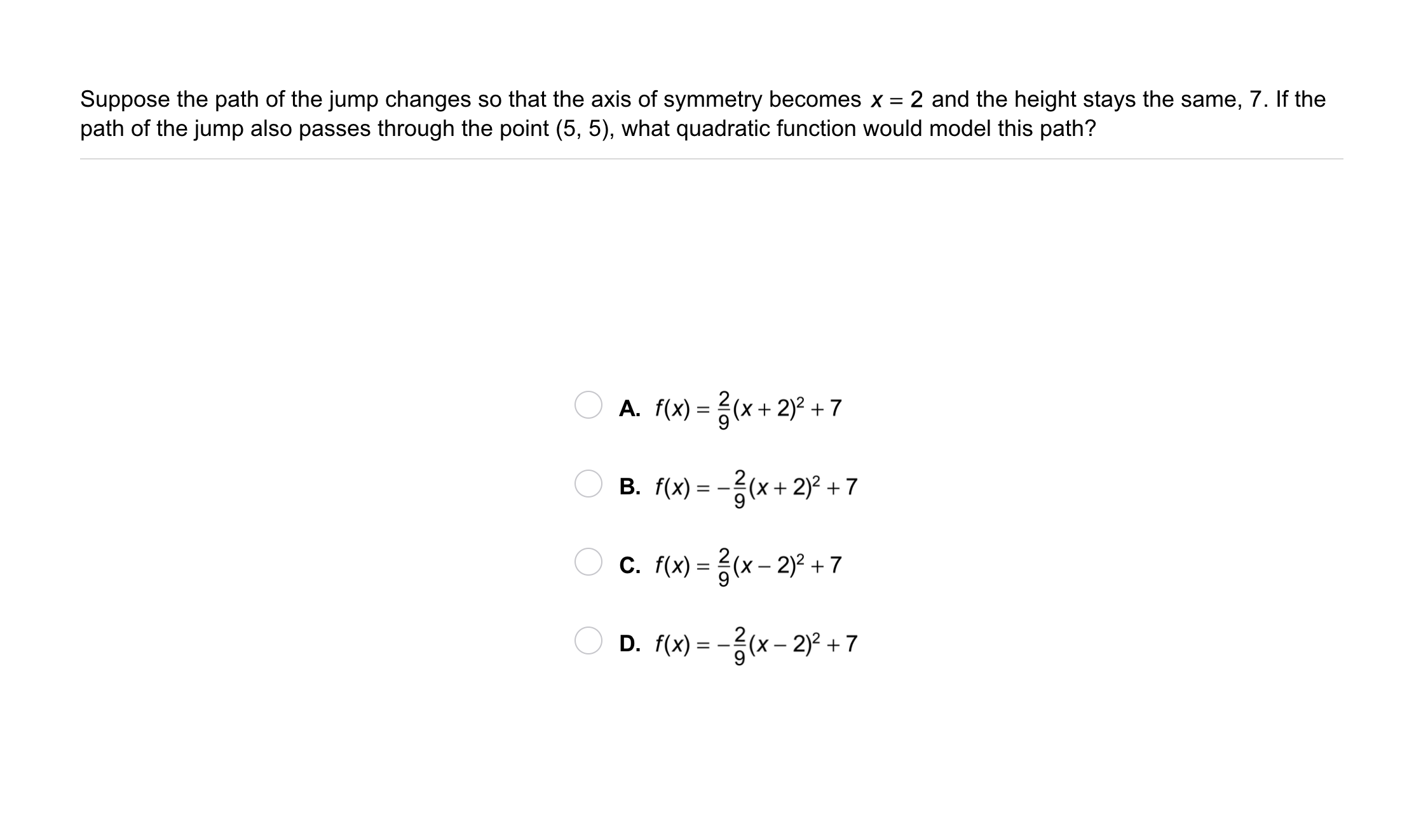11
11
10
Problem 5 Got It? Suppose the path of the jump changes so that the axis of symmetry becomes x = 2 and the height stays the same, 7. If the path of the jump also passes through the point (5, 5), what quaratic function would model this path?
A
B
C
D